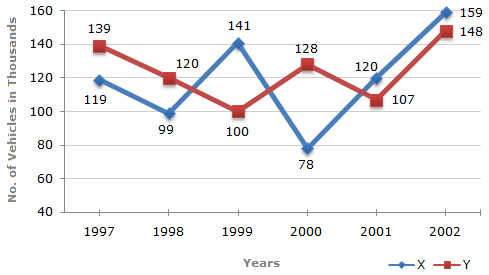# Data Interpretation - Line Charts - Discussion

### Discussion :: Line Charts - Line Chart 3 (Q.No.1)

Study the following line graph and answer the questions based on it.

Number of Vehicles Manufactured by Two companies ove the Years (Number in Thousands)1.

What is the difference between the number of vehicles manufactured by Company Y in 2000 and 2001 ?

 [A]. 50000 [B]. 42000 [C]. 33000 [D]. 21000

Answer: Option D

Explanation:

Required difference = (128000 - 107000) = 21000.

 Leki said: (Aug 27, 2011) What is required difference actually.? My answer would be Y=(128+107=235)-X=(78+120=198) 235-198=37... its 37000.

 Swetha said: (Feb 4, 2015) In 2000 company Y production z 128K where as in 2001 its production z 107k. So the difference between the number of vehicle production z 128000-107000 = 21000. i.e. 21k.

 Dezang said: (May 7, 2020) The answer should be B, as the question says difference between company Y in 2000 and 2001 i.e. 120-78.

#### Post your comments here:

Name *:

Email   : (optional)

» Your comments will be displayed only after manual approval.

#### Current Affairs 2021

Interview Questions and Answers